# About the Gaussian distribution of particles

Dear Fluka Expert,
I am having a problem learning source_newgen.f.
I want to verify my learning through the implementation of Gaussian distribution. First of all, I set the fwhm of x and y in the BEAM card, as shown in Figure 2, to emit protons from -38.95cm to the positive direction of the Z axis without setting the emitter separately. After a period of particle transport, Fig. 1 is obtained, which is a two-dimensional diagram of proton fluence at z=-22.5cm.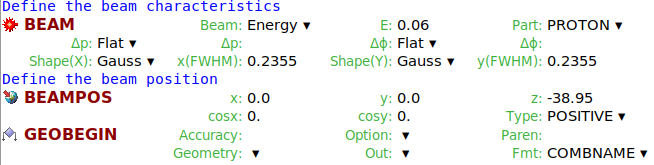Then, as a comparison, I modified the parameters in first.f.
first.f (18.8 KB)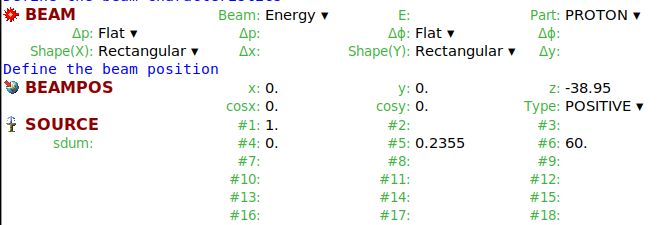``````  After compiling and running, I got Figure 3, which is also the proton flux at -22.5cm.
``````

Obviously, the results are very different, so I have the following questions: ① Is the particle Gaussian distribution implemented by the BEAM card different from that implemented by the code?Does the code implement a two-dimensional Gaussian distribution?② Why is the BEAM card required to set the fwhm of X and Y instead of the mean?And the code has to define the mean and fwhm, right?What’s the difference I don’t know about?③ Why can’t the FUNCTION option be checked on the BEAM card? The purpose of my study is to customize the probability density f(x,y).

Dear @luo.luo ,

There is an error in your user routine. By mistake you set the beam energy twice:
First in section 3.1 (this is what you expect), but you overwrite it with point 3.4.2 with a Gaussian energy sampling.

The beam divergence has to be set in section 4.1 and 4.2. Here you only need to the the fwhm of the (full opening) angle, as on the BEAM card, with the advantage, that you can specify different divergences in the X and Y planes. The divergences are always sampled around the beam direction, so no mean value is required.

Since you didn’t set divergence values in your user routine, you get a pencil beam, which explains the difference between your plots.

Unfortunately I’m not familiar with the Function option in Flair. @vasilis could you explain this feature?

Cheers,
David

1 Like

In the BEAM what(1) you’ve assign a function e.g.

``````#define EKIN 1.0
BEAM p: =EKIN
``````

flair will try to evaluate the function and if positive it will show that it is Momentum you are setting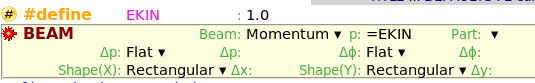if the evaluation gives a negative number it will select the Energy.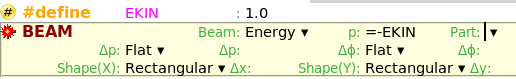However if for some reason flair cannot determine the value, because let say the #define is not active (but will be activated latter for a run) flair will show Function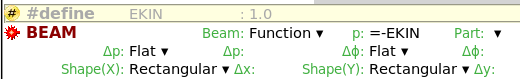So the Function is not something you can manually select. Flair selects it automatically if it cannot figure out the result

2 Likes